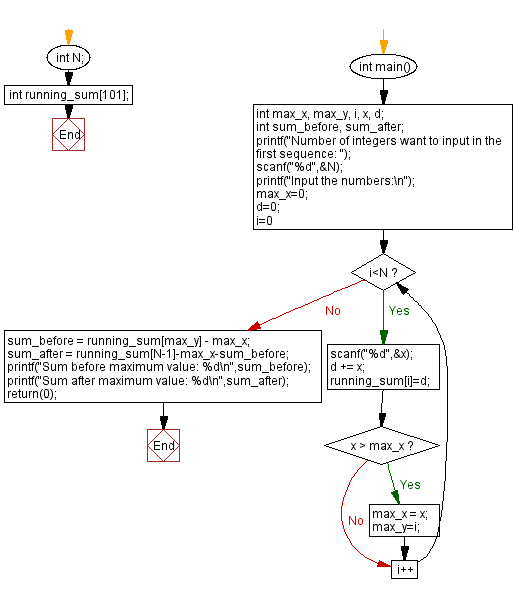﻿ C - Sum values before, after the maximum value in a sequence

# C Exercises: Sum values before, after the maximum value in a sequence

## C Basic-II: Exercise-5 with Solution

Write a C program that accept a sequence of different values and calculate the sum of the values before, after the maximum value.
The sum of the values before the maximum value is 0, if there are no values before the maximum. Similarly, the sum of the values after the maximum value is 0, if there are no values after the maximum.

Sample Date:

1 2 3 -> 3 0
1 2 9 4 5 -> 3 9
2 2 2 2 -> 0 6

C Code:

``````#include <stdio.h>
int N;
int running_sum;
int main()
{
int max_x, max_y, i, x, d;
int sum_before, sum_after;

printf("Number of integers want to input in the first sequence: ");
scanf("%d",&N);
printf("Input the numbers:\n");
max_x=0;
d=0;
for(i=0;i<N;i++)
{
scanf("%d",&x);
d += x;
running_sum[i]=d;
if(x > max_x)
{
max_x = x;
max_y=i;
}
}
sum_before = running_sum[max_y] - max_x;
sum_after = running_sum[N-1]-max_x-sum_before;
printf("Sum before maximum value: %d\n",sum_before);
printf("Sum after maximum value: %d\n",sum_after);
return(0);
}
``````

Sample Output:

```Number of integers want to input in the first sequence: 5
Input the numbers:
1 2 9 4 5
Sum before maximum value: 3
Sum after maximum value: 9
```

Flowchart:C Programming Code Editor:

Previous C Programming Exercise: Common element(s) of two sequences.
Next C Programming Exercise: Length of longest ascending contiguous subsequence.

What is the difficulty level of this exercise?

Test your Programming skills with w3resource's quiz.

﻿

```MY_TYPE a = { .flag = true, .value = 123, .stuff = 0.456 };